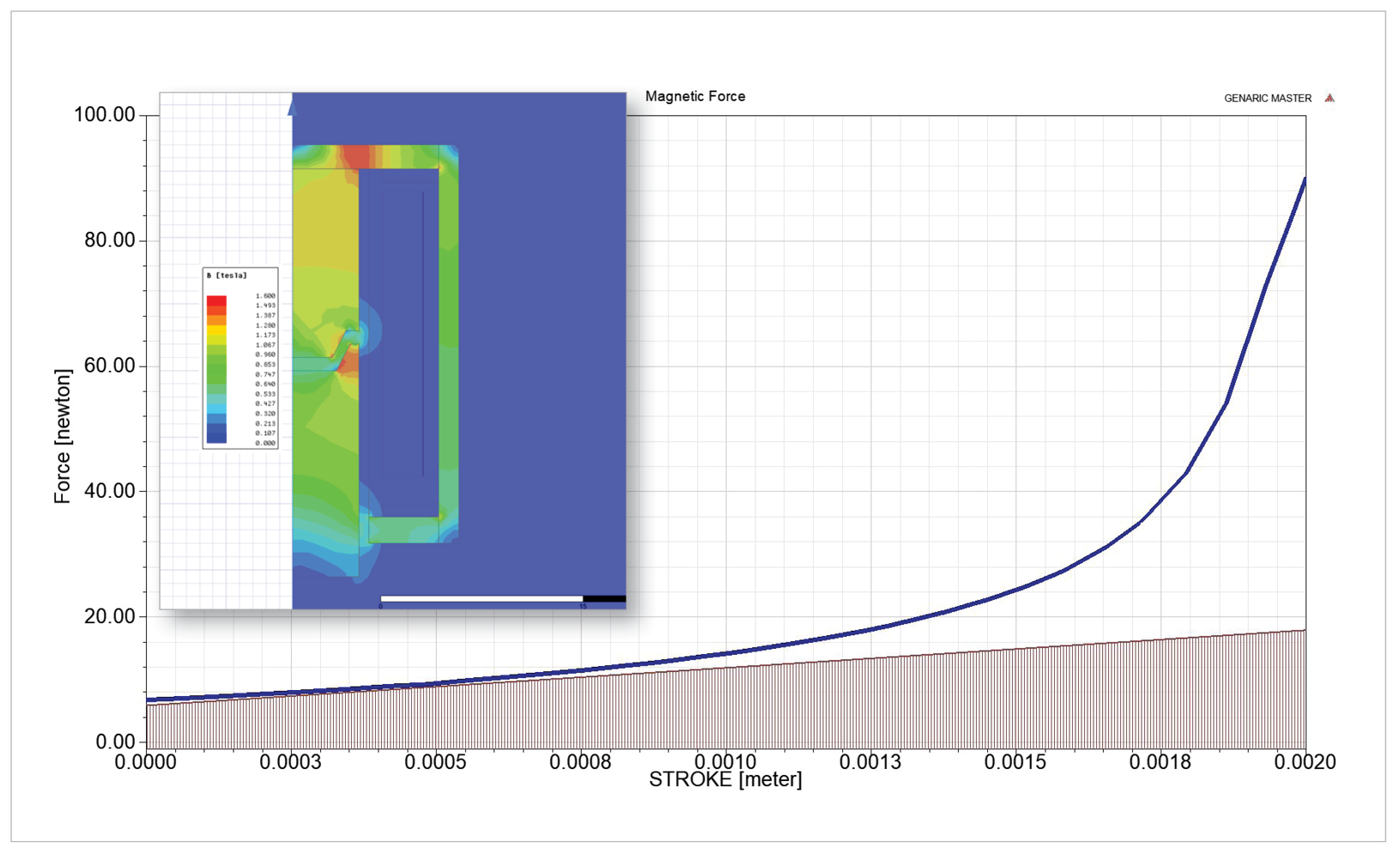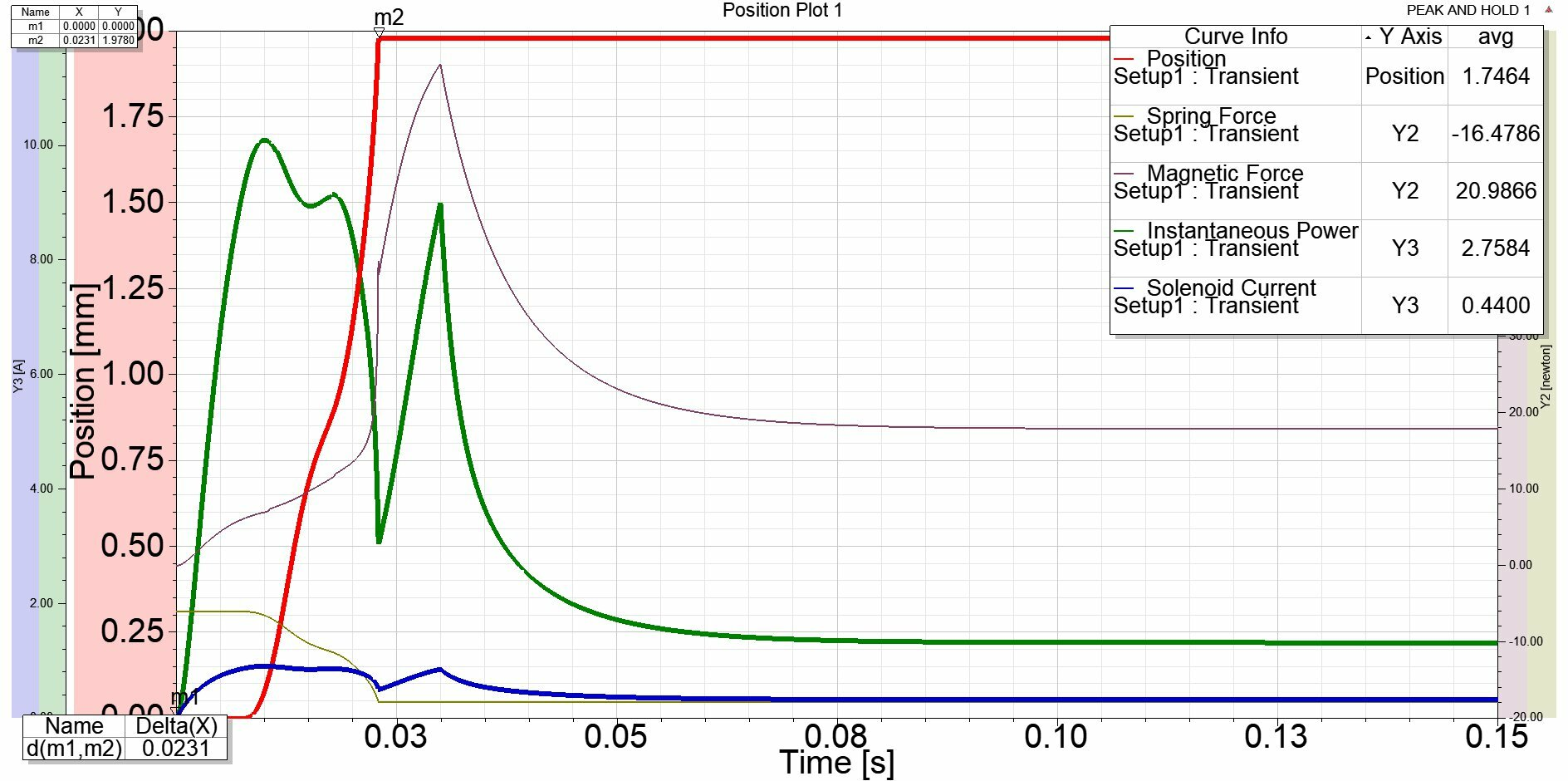Now Hiring:TLX Technologies is hiring a Quality Technician to join our growing Quality team!

# Energy Efficiency: Optimizing Power Consumption in Solenoid Operation

October 22, 2021

The continued push for electrification, as well as the adoption of alternative energy sources, is transforming system and component design in all types of vehicles. In the search for advanced battery technologies and more intelligent energy usage, the systems and components under development that will meet the challenges of current and future vehicle platforms will require efficient use of every joule of energy.

While solenoids and solenoid valves are proven, long-standing staples in converting electrical energy to work, one of the key characteristics that often gets lost in the specification details is the force versus position curve of the linear electromagnetic actuator. As the solenoid plunger moves closer and closer to the pole of the solenoid, force increases exponentially. For example, if a solenoid needs to compress a spring 2 millimeters from a preload height and hold it there for 150 milliseconds, the preload is 6 newtons, and the spring’s rate is 6 newtons/millimeter. This would mean that 0.024 newton meters of work need to be performed (W=F x d). This is a convenient since, in the context of units of work, the Newton meter is equal to the joule.

### Figure 1As the plunger moves closer to the pole, the level of force required increases exponentially.

As can be seen from the force curve (Figure 1), there is just enough force to get the job done over the first millimeter of travel. Over the second millimeter of travel, the force becomes much significantly higher than what is necessary, making it seem like this solenoid is inefficient in this application. In fact, the solenoid outputs 0.42 joules of work, almost double what is needed. The transient simulation of the actuation cycle reveals a compounding of this inefficiency because the position must be held (offer hold force without providing additional work) for an additional period. In all, 1.64 joules of energy are used during the 150-millisecond cycle to do 0.024 joules of work.

### Control Strategy

This inefficiency is simply the way electromagnetic force works. However, this waste can be mitigated by designing an appropriate control strategy for the solenoid valve. This is commonly known as peak-and-hold. Since 90 newtons of force is achieved at the full stroke of the solenoid, the input voltage for the hold cycle can be reduced. Looking at the transient model of the solenoid (Figure 2), the spring is compressed and full stroke is reached within 22 milliseconds. This means that in the initial simulation, 1.22 joules are spent just holding the solenoid in place.

With a peak-and-hold control strategy, the hold voltage can be reduced to 4 volts or 33% of the voltage needed to accomplish the initial actuation and still accomplish the task of holding the spring compressed. For this example, 12 volts is provided for 30 milliseconds to accomplish the initial pull-in. The voltage is then dropped to 4 Vdc for the remainder of the 150-millisecond cycle. Shown by the instantaneous power trace (green) in the transient model (Figure 2), the energy used is more closely matched to the energy needed to complete the task. The net result is a total energy consumption of 0.41 joules—a 75% improvement.

### Figure 2Peak-and-hold control strategy simulation. This shows that significant gains in efficiency can be made by selecting the right control strategy.

The peak-and-hold control strategy is an ideal solution to mitigate the inherent inefficiencies of electromagnetic solenoids, allowing the same solenoid to do the same amount of work using far less energy. In addition, this is a great reminder for manufacturers to look beyond the specifications to leverage historic or current technologies in new ways to meet critical goals such as making gains in energy efficiency.